﻿ 石墨烯内聚能的密度泛函理论研究 DFT Studies on the Cohesive Energy of Graphene

Applied Physics
Vol. 11  No. 01 ( 2021 ), Article ID: 39791 , 8 pages
10.12677/APP.2021.111001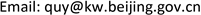DFT Studies on the Cohesive Energy of Graphene

Yan Qu

Beijing Advanced Materials and New Energy Technology Development Center, BeijingReceived: Dec. 11th, 2020; accepted: Jan. 11th, 2021; published: Jan. 18th, 2021ABSTRACT

In this paper, the geometric structure and cohesive energy of graphene are investigated under local density approximation (LDA) as well as generalized gradient approximation (GGA) based on first principles and density functional theory. Graphene is a hexagonal structure composed of carbon atoms. The total energy of the graphene system and the total energy of the isolated carbon atom system are calculated by the density functional theory. The calculation results show that the cohesive energy of graphene is about -9 to -10eV, and for different calculation methods and parameters, the cohesive energy obtained is always negative, which indicates that the graphene structure has lower energy relative to the isolated carbon atoms. Therefore, the attractive interaction makes the system more inclined to form the graphene structure compared to the isolated carbon atoms, indicating that the graphene structure is stable. This computational simulation study can provide a theoretical reference for the future development of graphene structures, as well as the performance studies and applications of novel structures (e.g., twist graphene).

Keywords:First Principles, Density Functional Theory, Graphene, Cohesive Energy1. 引言

2004年，英国学者Geim和Novoselov  发现他们可以用一种很简易的方式获得愈来愈薄的石墨薄片。他们通过从高定向热解石墨中剥离出石墨片，然后将薄片的两面粘在胶带上面，进一步撕开胶带从而将石墨一分为二。正是通过不断地这样操作，最终可以得到仅由一层碳原子构成的石墨烯结构。石墨烯是一种由碳原子组成六角型呈蜂巢晶格的二维材料 ，由于其具有优良的光学、力学、电学性能以及丰富的物理特性，在物理、材料学、加工、能源、医学等领域有着广阔的应用前景   ，近些年来一直是人们研究的热点。

2. 计算方法及过程

2.1. 理论方法

$E\left[\rho \right]={T}_{0}\left[\rho \right]+{U}_{ext}\left[\rho \right]+{E}_{cl}\left[\rho \right]+{E}_{xc}\left[\rho \right]$ (1)

$\rho \left(r\right)={\sum }_{i=1}^{N}{|{\varphi }_{i}^{KS}\left(r\right)|}^{2}$ (2)

$\left[-\frac{1}{2}{\nabla }_{i}^{2}+{\stackrel{^}{V}}_{eff}\left(r\right)\right]{\varphi }_{i}^{KS}\left(r\right)={ϵ}_{i}{\varphi }_{i}^{KS}\left(r\right)$ (3)

$E\left[\rho \right]={\sum }_{i=1}^{N}{ϵ}_{i}-\frac{1}{2}\iint \frac{\rho \left(r\right)\rho \left({r}^{\prime }\right)}{|r-{r}^{\prime }|}\text{d}r\text{d}{r}^{\prime }-\int {\stackrel{^}{V}}_{xc}\left(r\right)\rho \left(r\right)\text{d}r+{E}_{xc}\left[\rho \right]$ (4)

${\sum }_{{G}^{\prime }}\left[\frac{1}{2}{|k+{G}^{\prime }|}^{2}{\delta }_{G{G}^{\prime }}+{V}_{kG{G}^{\prime }}^{eff}\right]{\varphi }_{j}\left({G}^{\prime }\right)={ϵ}_{j}{\varphi }_{j}\left(G\right)$ (5)

$\begin{array}{c}{E}_{tot}=\underset{j}{\sum }{ϵ}_{j}-\Omega \frac{1}{2}\underset{G\ne 0}{\sum }{V}_{coul}\left(G\right){\rho }^{*}\left(G\right)-\Omega \underset{G\ne 0}{\sum }\left[{V}_{xc}\left(G\right)-{ϵ}_{xc}\left(G\right)\right]{\rho }^{*}\left(G\right)\\ \text{\hspace{0.17em}}\text{\hspace{0.17em}}+{\sum }_{\nu }{\alpha }_{\nu }{\sum }_{\mu }{Z}_{\mu }+{\gamma }_{Ewald}\end{array}$ (6)

$\frac{{\hslash }^{2}}{2m}{|k+G|}^{2}\le {E}_{cutoff}$ (7)

2.2. 计算石墨烯的几何结构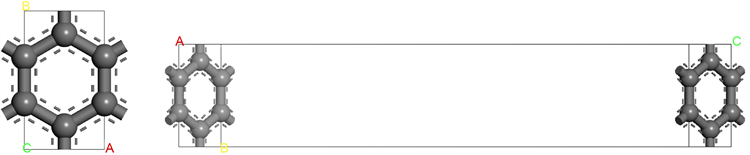Figure 1. Schematic diagram of the structure of graphene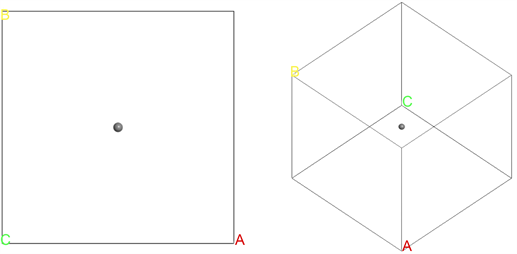Figure 2. Schematic diagram of the structure of isolated carbon atom

2.2. 计算过程及参数的选取

2.2.1. 石墨烯体系计算Table 1. Parameters of graphene total energy calculation

2.2.2. 孤立碳原子体系计算Table 2. Parameters of isolated carbon atom total energy calculation

3. 计算结果和分析

$\text{Finalenergy},E=-621.8196941698\text{\hspace{0.17em}}\text{eV}$ (8)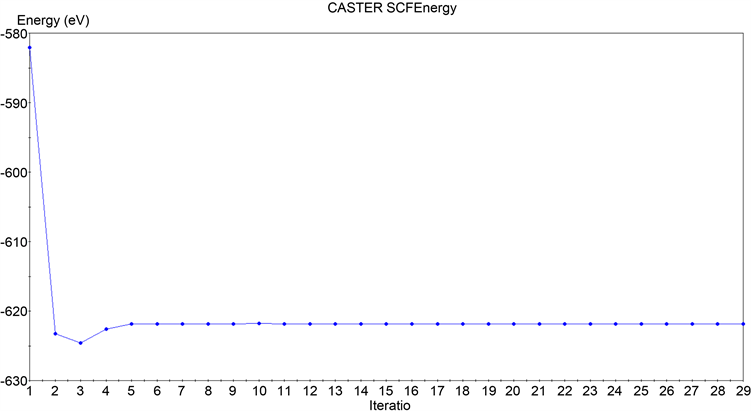Figure 3. Convergence results of total graphene energy calculation

$\text{Finalenergy},E=-145.3495145913\text{\hspace{0.17em}}\text{eV}$ (9)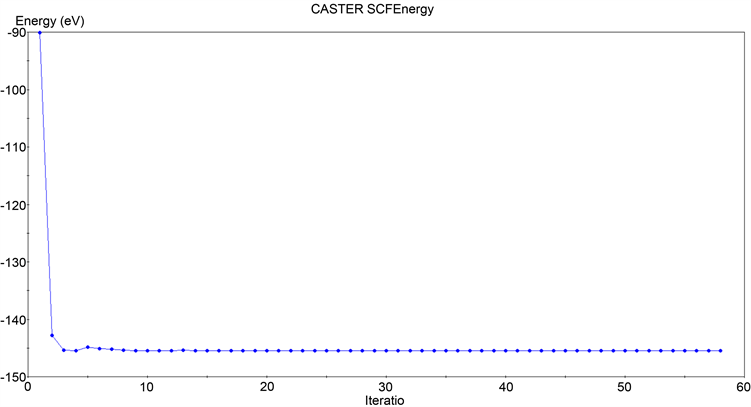Figure 4. Convergence results of total isolated carbon atom energy calculation

$E=\frac{-621.8196941698\text{\hspace{0.17em}}\text{eV}-\left(-4×145.3495145913\text{\hspace{0.17em}}\text{eV}\right)}{4}\approx -10.105\text{\hspace{0.17em}}\text{eV}$ (10)

$\text{Finalenergy},E=-620.0817119187\text{\hspace{0.17em}}\text{eV}$ (11)

$E=\frac{-620.0817119187\text{\hspace{0.17em}}\text{eV}-\left(-4×145.3500139220\text{\hspace{0.17em}}\text{eV}\right)}{4}\approx -9.670414\text{\hspace{0.17em}}\text{eV}$ (12)

Table 3. Calculation of the total energy of graphene under different parameters and methodsTable 4. Calculation of the total energy of isolated carbon atom under different parameters and methods

4. 结果分析与讨论

DFT Studies on the Cohesive Energy of Graphene[J]. 应用物理, 2021, 11(01): 1-8. https://doi.org/10.12677/APP.2021.111001

1. 1. Geim, A.K. and Novoselov, K.S. (2009) The Rise of Graphene. Nature Materials, 6, 11-19. https://doi.org/10.1142/9789814287005_0002

2. 2. 徐秀娟, 秦金贵, 李振. 石墨烯研究进展[J]. 化学进展, 2009, 21(12): 2559-2567.

3. 3. 黄毅, 陈永胜. 石墨烯的功能化及其相关应用[J]. 中国科学B辑: 化学, 2009, 39(9): 887-896.

4. 4. 张文毓, 全识俊. 石墨烯应用研究及进展[J]. 传感器世界, 2011, 17(5): 6-11.

5. 5. 吕鹏, 冯奕钰, 张学全, 等. 功能化石墨烯的应用研究新进展[J]. 中国科学: 技术科学, 2010(11): 1247-1256.

6. 6. 黎乐民, 刘俊婉, 金碧辉. 密度泛函理论[J]. 中国基础科学, 2007, 7(3): 27-28.

7. 7. Newton, M.D. and Sutin, N. (1984) Electron Transfer Reactions in Condensed Phases. Annual Review of Physical Chemistry, 35, 437-480. https://doi.org/10.1146/annurev.pc.35.100184.002253

8. 8. 李震宇, 贺伟, 杨金龙. 密度泛函理论及其数值方法新进展[J]. 化学进展, 2005, 17(2): 192-202.

9. 9. Hohenberg, P. and Kohn, W. (1964) Inhomogeneous Electron Gas. Physical Review, 136, B864. https://doi.org/10.1103/PhysRev.136.B864

10. 10. Cococcioni, M. and de Gironcoli, S. (2005) Linear Response Ap-proach to the Calculation of the Effective Interaction Parameters in the LDA+U Method. Physical Review B, 71, Article ID: 035105. https://doi.org/10.1103/PhysRevB.71.035105

11. 11. Kohn, W. and Sham, L. (1965) Self-Consistent Equations In-cluding Exchange and Correlation Effects. Physical Review, 140, A1133. https://doi.org/10.1103/PhysRev.140.A1133

12. 12. Troullier, N. and Martins, J.L. (1991) Efficient Pseudopotentials for Plane-Wave Calculations. Physical Review B, 43, 1993-2006. https://doi.org/10.1103/PhysRevB.43.1993

13. 13. Lin, J.S., Qteish, A., Payne, M.C. and Heine, V. (1993) Optimized and Transferable Nonlocal Separable Ab Initio Pseudopotentials. Physical Review B, 47, 4174-4180. https://doi.org/10.1103/PhysRevB.47.4174

14. 14. Hamann, D.R., Schluter, M. and Chiang, C. (1979) Norm-Conserving Pseudopotentials. Physical Review Letters, 43, 1494-1497. https://doi.org/10.1103/PhysRevLett.43.1494

15. 15. Vanderbilt, D. (1990) Soft Self-Consistent Pseudopotentials in a Generalized Eigenvalue Formalism. Physical Review B, 41, 7892-7895. https://doi.org/10.1103/PhysRevB.41.7892

16. 16. Perdew, J.P., Burke, K. and Ernzerhof, M. (1996) Generalized Gradient Approximation Made Simple. Physical Review Letters, 77, 3865-3868. https://doi.org/10.1103/PhysRevLett.77.3865